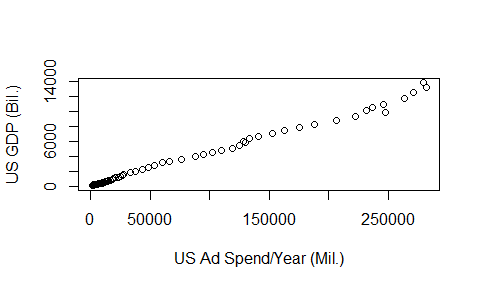# Lecture 10: Relationships Between Variables, Part 2

## Recap

• Last time we talked about how to think about the relationship between two variables
• We talked about dependence and correlation
• As illustrated using proportion tables (`prop.table`), differences in means (`group_by() %>% summarize()`), correlation (`cor`), and graphically with scatterplots (`plot(xvar,yvar)`) and overlaid densities (`plot(density())` followed by `lines(density())`)

## Today

• We’re going to be going much further into explaining
• How can we use one variable to explain another and what does that mean?
• One way to think about what we’re doing is to translate “how does `X` explain `Y`” as “what would I expect `Y` to look like, given a certain value of `X`?”

## Explanation

• Why do we care?
• Explaining is a very flexible way of understanding the relationship between two variables
• Plus, it lets us put a magnitude on these relationships
• “How much of the variation in earnings is explained by education?”
• “How much of the variation in earnings is not explained by education?”

## Explanation

• Plus, this will end up being very important when we get to causality
• Think back to this graph from last time:
``````addata <- read.csv('http://www.nickchk.com/ad_spend_and_gdp.csv')
xlab='US Ad Spend/Year (Mil.)',ylab='US GDP (Bil.)')``````## Explanation

• We know that part of the reason for the relationship we see is inflation
• Explanation lets us say things like “not counting the parts of ad spend and GDP that are explained by inflation, what is the relationship between ad spend and GDP?”
• When we get into causality, this will let us isolate just the parts of the relationship we’re interested in

## Simple Explanation

• So that’s our goal - for different values of `X`, see what `Y` looks like.
• There are lots of ways to do this - one of which is called regression and you’ll see that in later classes
• In this class we’re going to focus on a very simple approach - simply taking the mean of `Y` for different values of `X`.

## Simple Explanation

• Basically, we’re trying to do a simpler version of this: